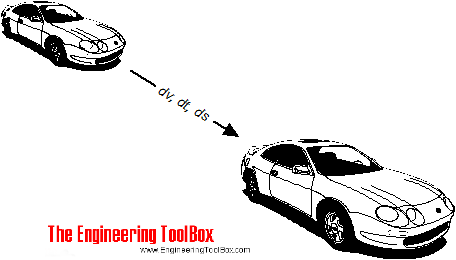Engineering ToolBox - Resources, Tools and Basic Information for Engineering and Design of Technical Applications!

# Impulse and Impulse Force

## Forces acting a very short time are called impulse forces.

According to Newton's Second Law of Motion - force can be expressed as

F = dM / dt                                      (1)

where

F = applied force (N, lbf)

dM = change of momentum (kg m/s, lb ft/s)

dt = time taken (s)

Eq. (1) can alternatively be expressed as

dM = F dt                                      (1b)

Change in momentum is force times acting time.

Forces that act for a very short time are called impulse forces. The product of the impulsive force and the time it acts is called the impulse. The impulse is equal to the change of momentum caused by the impulsive force and can be expressed as

I = F dt = dM                                      (2)

where

I = impulse (N s)

### Example - resulting Velocity after an Acting Force

A force 1000 N is acting on a car with mass 1000 kg in 10 second.The impulse - or change in momentum - can be calculated

I = dM = (1000 N) (10 s)

= 10000 (N s, kg m/s)

Since momentum can be expressed as

M = m v                                                 (3)

where

M = momentum (kg m/s, lb ft/s)

m = mass (kg, lb)

v = velocity (m/s, ft/s)

then the velocity of the car after 10 s can be calculated as

v = (10000 kg m/s) / (1000 kg)

= 10 m/s

= 36 km/h

## Related Topics

• ### Dynamics

Motion - velocity and acceleration, forces and torque.
• ### Mechanics

Forces, acceleration, displacement, vectors, motion, momentum, energy of objects and more.

## Related Documents

• ### Angular Motion - Power and Torque

Angular velocity and acceleration vs. power and torque.
• ### Car - Required Power and Torque

Power, torque, efficiency and wheel force acting on a car.
• ### Conservation of Momentum

The momentum of a body is the product of its mass and velocity - recoil calculator.
• ### Distance Traveled vs. Speed and Time - Calculator and Chart

Velocity plotted in time used diagram.
• ### Flywheels - Kinetic Energy

The kinetic energy stored in flywheels - the moment of inertia.
• ### Force Ratio

The force ratio is the load force versus the effort force.
• ### Formulas of Motion - Linear and Circular

Linear and angular (rotation) acceleration, velocity, speed and distance.
• ### Impact Force

Impact forces acting on falling objects hitting the ground, cars crashing and similar cases.
• ### Jet Propulsion

Calculate the propulsive discharge force or thrust induced by an incompressible jet flow.
• ### Kinetic Energy

Energy possessed by an object's motion is kinetic energy.
• ### Projectile Range

Calculate the range of a projectile - a motion in two dimensions.
• ### Rolling Resistance

Rolling friction and rolling resistance.
• ### Support Reactions - Equilibrium

Static equilibrium is achieved when the resultant force and resultant moment equals to zero.

## Engineering ToolBox - SketchUp Extension - Online 3D modeling!

Add standard and customized parametric components - like flange beams, lumbers, piping, stairs and more - to your Sketchup model with the Engineering ToolBox - SketchUp Extension - enabled for use with older versions of the amazing SketchUp Make and the newer "up to date" SketchUp Pro . Add the Engineering ToolBox extension to your SketchUp Make/Pro from the Extension Warehouse !

We don't collect information from our users. More about

## Citation

• The Engineering ToolBox (2008). Impulse and Impulse Force. [online] Available at: https://www.engineeringtoolbox.com/impulsive-forces-d_1376.html [Accessed Day Month Year].

Modify the access date according your visit.

9.19.12